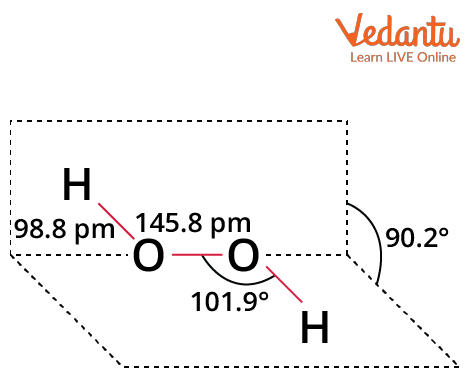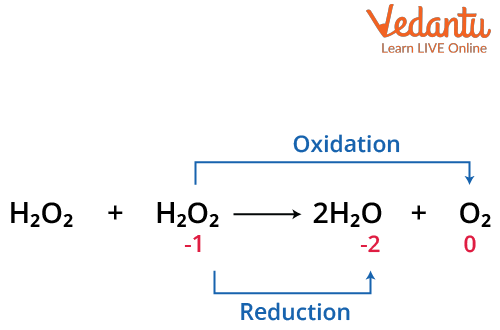# Reducing and Oxidising Nature of Hydrogen Peroxide for JEE## Introduction to Hydrogen Peroxide

With the chemical formula H2O2, hydrogen peroxide is a substance that is frequently used in the chemistry laboratory. It has a characteristic pale blue colour in pure liquid form. However, it appears as a colourless combination with a mildly pungent odour in a diluted solution at ambient temperature.

When it is dissolved in water, it does not completely ionise. The hydrogen peroxide solution is a weak acid often sold in concentrations of 30, 50, and 70% by weight. H2O2 has a slightly higher viscosity than water. Small amounts of gaseous hydrogen peroxide, produced when UV rays interact with oxygen in the presence of moisture, are naturally present in the air. The respiratory tract is irritated by hydrogen peroxide vapour. It has chemical and physical properties and advantages and disadvantages, which are further discussed below.

## Preparation of Hydrogen Peroxide

There are two techniques to make hydrogen peroxide:

• Laboratory Procedure
When barium peroxide (BaO2)  is acidified, the excess water is removed by evaporation under reduced pressure and the result is hydrogen peroxide.

$\mathrm{H}_{2}\mathrm{SO}_{4}(\mathrm{aq})+\mathrm{BaO}_{2}(\mathrm{~s})=\mathrm{BaSO}_{4}(\mathrm{~s})+\mathrm{H}_{2} \mathrm{O}_{2}(\mathrm{aq})+8 \mathrm{H}_{2} \mathrm{O}(\mathrm{l})$

• Industrial Approach
Hydrogen peroxide is created by electrolyzing ice-cold H2SO4 by 30%. An acidified sulphate solution is electrolyzed at a high current density to create peroxodisulphate. Hydrogen peroxide is produced via the hydrolysis of peroxodisulphate.

$2 \mathrm{HSO}_{4}^{-}(\mathrm{aq}) \rightarrow \mathrm{S}_{2} \mathrm{O}_{8}^{-2}(\mathrm{aq}) \rightarrow 2 \mathrm{HSO}_{4}^{-}(\mathrm{aq})+2 \mathrm{H}^{+}+\mathrm{H}_{2} \mathrm{O}_{2}(\mathrm{aq})$

## Structure of Hydrogen PeroxideStructure of Hydrogen Peroxide

Hydrogen peroxide has a non-planar structure and an open book structure. 111o is the dihedral angle. O-O bonds have a length of 145.8 pm, while O-H bonds have a length of 98.8 pm (10-13 m). This structure consists of two planes, each having an O-H bond pair. The angle between the two planes is 90.2o.

Due to the bent molecular geometry of H2O2 and the existence of a lone pair on its oxygen core atom, there is an uneven distribution of charges. Therefore, the molecule is polar.

## Oxidising and Reducing Properties of Hydrogen Peroxide

Now we will explain any two oxidising and reducing properties of hydrogen peroxide.

### Reducing Nature of Hydrogen Peroxide

Towards strong oxidising agents, H2O2 is a reducing agent in reaction.

• In Acidic Medium:

Basic reaction: $-\mathrm{H}_{2} \mathrm{O}_{2} \rightarrow \mathrm{O}_{2}+2 \mathrm{H}^{+}+2 \mathrm{e}^{-}$

1. $2 \mathrm{KMnO}_{4}+3 \mathrm{H}_{2} \mathrm{SO}_{4}+5 \mathrm{H}_{2} \mathrm{O} \rightarrow \mathrm{K}_{2} \mathrm{SO}_{4}+2 \mathrm{MnSO}_{4}+5 \mathrm{H}_{2} \mathrm{O}$

2. $\mathrm{K}_{2} \mathrm{Cr}_{2} \mathrm{O}_{7}+4 \mathrm{H}_{2} \mathrm{SO}_{4}+3 \mathrm{H}_{2} \mathrm{O}_{2} \rightarrow \mathrm{K}_{2} \mathrm{SO}_{4}+\mathrm{Cr}_{2}\left(\mathrm{SO}_{4}\right)_{4}+7 \mathrm{H}_{2} \mathrm{O}+3 \mathrm{O}_{2}$

• In Alkaline Medium:

Basic reaction $-\mathrm{H}_{2} \mathrm{O}_{2}+2 \mathrm{OH}^{-} \rightarrow 2 \mathrm{H}_{2} \mathrm{O}+\mathrm{O}_{2}+2 \mathrm{e}^{-}$

1. $2 \mathrm{~K}_{3} \mathrm{Fe}(\mathrm{CN})_{6}+2 \mathrm{KOH}+\mathrm{H}_{2} \mathrm{O}_{2} \rightarrow 2 \mathrm{~K}_{4} \mathrm{Fe}(\mathrm{CN})_{6}+2 \mathrm{H}_{2} \mathrm{O}+\mathrm{O}_{2}$

2. $\mathrm{Fe}_{2}\left(\mathrm{SO}_{4}\right)_{3}+2 \mathrm{KOH} \rightarrow \mathrm{H}_{2} \mathrm{O}_{2} \rightarrow 2 \mathrm{FeSO}_{4}+\mathrm{K}_{2} \mathrm{SO}_{4}+2 \mathrm{H}_{2} \mathrm{O}+\mathrm{O}_{2}$

Oxidising Properties of Hydrogen Peroxide

In both acidic and alkaline environments, H2O2 functions as an oxidising agent.

• In Acid Solution:

Basic reaction; $\mathrm{H}_{2} \mathrm{O}_{2}+2 \mathrm{H}^{+}+2 \mathrm{e}^{-} \rightarrow 2 \mathrm{H}_{2} \mathrm{O}$

1. $\mathrm{PbS}+4 \mathrm{H}_{2} \mathrm{O}_{2} \rightarrow \mathrm{PbSO}_{4}+4 \mathrm{H}_{2} \mathrm{O}$

2. $2 \mathrm{KI}+\mathrm{H}_{2} \mathrm{SO}_{4}+\mathrm{H}_{2} \mathrm{O}_{2} \rightarrow \mathrm{K}_{2} \mathrm{SO}_{4}+2 \mathrm{H}_{2} \mathrm{O}+\mathrm{I}_{2}$

3. $\mathrm{H}_{2} \mathrm{~S}+\mathrm{H}_{2} \mathrm{O}_{2} \rightarrow 2 \mathrm{H}_{2} \mathrm{I}+\mathrm{S}$

• Alkaline Solution:

Basic reaction; $\mathrm{H}_{2} \mathrm{O}_{2}+2 \mathrm{OH}^{-}+2 \mathrm{e}^{-} \rightarrow 2 \mathrm{H}_{2} \mathrm{O}+2 \mathrm{O}^{2-}$

1. $2 \mathrm{HCHO}+\mathrm{H}_{2} \mathrm{O}_{2} \rightarrow 2 \mathrm{HCOOH}+\mathrm{H}_{2}$

## Physical Properties of Hydrogen Peroxide

Clear and colourless hydrogen peroxide is totally miscible with water. A variety of organic solvents, including carboxylic esters, can dissolve hydrogen peroxide and its extremely concentrated aqueous solutions. Distillation can totally separate hydrogen peroxide and water since they don't mix to generate an azeotropic combination. It melts at 272.4 K and has a boiling point of 423 K (extrapolated). It has a faintly unpleasant odour. Aqueous solution has a density of 1.11 $\dfrac{g}{cc}$, while pure form has a density of 1.450 $\dfrac{g}{cc}$.

## Chemical Properties of Hydrogen Peroxide

1. Decomposition: H2O2 decomposes on standing or on heating.

$\mathrm{H}_{2} \mathrm{O}_{2}+\mathrm{H}_{2} \mathrm{O}_{2} \rightarrow 2 \mathrm{H}_{2} \mathrm{O}+\mathrm{O}_{2}$

Note: This is catalysed by Pt, Ag, Co, Fe, Cu, MnO2 etc.Decomposition of Hydrogen Peroxide

1. Bleaching Action: Acts as a mild bleaching agent due to the oxidation reaction.

$\mathrm{H}_{2} \mathrm{O}_{2} \rightarrow \mathrm{H}_{2} \mathrm{O}+[\mathrm{O}]$

$\mathrm{CH}_{2}=\mathrm{CH}_{2}+\mathrm{H}_{2} \mathrm{O}_{2} \rightarrow \mathrm{HOH}_{2} \mathrm{C}-\mathrm{CH}_{2} \mathrm{OH}$

## Conclusion

One of the safest and most useful compounds is hydrogen peroxide. Due to its advantageous characteristics, hydrogen peroxide is used in a wide range of application areas, including aseptic packaging as a sterilising agent; cosmetics and medicine as an antimicrobial agent; chemical synthesis as a potent and environmentally friendly oxidising agent; pulp, paper, and textile industry as a versatile bleaching agent; and transportation, where highly concentrated, highly pure hydrogen peroxide is used as a propellant. Due to its beneficial characteristics, hydrogen peroxide is the subject of numerous investigations. Although this chemical has a wide range of uses, its consumption is still very small. High production costs are the primary cause of this. Also, it is chemically unstable at lower concentrations.

## FAQs on Reducing and Oxidising Nature of Hydrogen Peroxide for JEE

1. What are the uses of hydrogen peroxide?

The following are the uses of hydrogen peroxide:

• Hydrogen peroxide has chemical applications, biological function, domestic, and therapeutic uses, including use as antimicrobial and oxidising agent.
• Hydrogen peroxide is used in municipal wastewater applications and cost-effective control of hydrogen sulphide in municipal sludge. It is also used for industrial applications such as pulp and paper bleaching.
• Hydrogen peroxide has been used for sulphide oxidation, for high-strength wastewater pretreatment, and also to reduce the biochemical oxygen demand (BOD) and chemical oxygen demand (COD) of industrial wastewaters.

2. What are the disadvantages of hydrogen peroxide?

Although certain side effects of hydrogen peroxide may occur, these reactions often do not require medical attention. These adverse effects may diminish throughout treatment as our body adjusts to the medication. We can consult a doctor if any of the following side effects last or become bothersome, or if we have any concerns about them like skin discoloration, peeling or crusted skin, natural skin colour lightening, or lightening of dark-skinned areas that have been treated. These are the following disadvantages of hydrogen peroxide (H2O2).

3. What is the molecular geometry of hydrogen peroxide (H2O2)?

The answer is that the molecule has twisted geometry and is not planar. It is also claimed to have an open book structure. According to the VSEPR theory, lone pair of electrons on oxygen atoms will try to repel the electron cloud of another atom as little as possible to minimize the repulsion. As a result, unbonded electron pairs push the hydrogen atoms that are next to it down and stretch the structure, giving it the appearance of an open book structure.

Comment